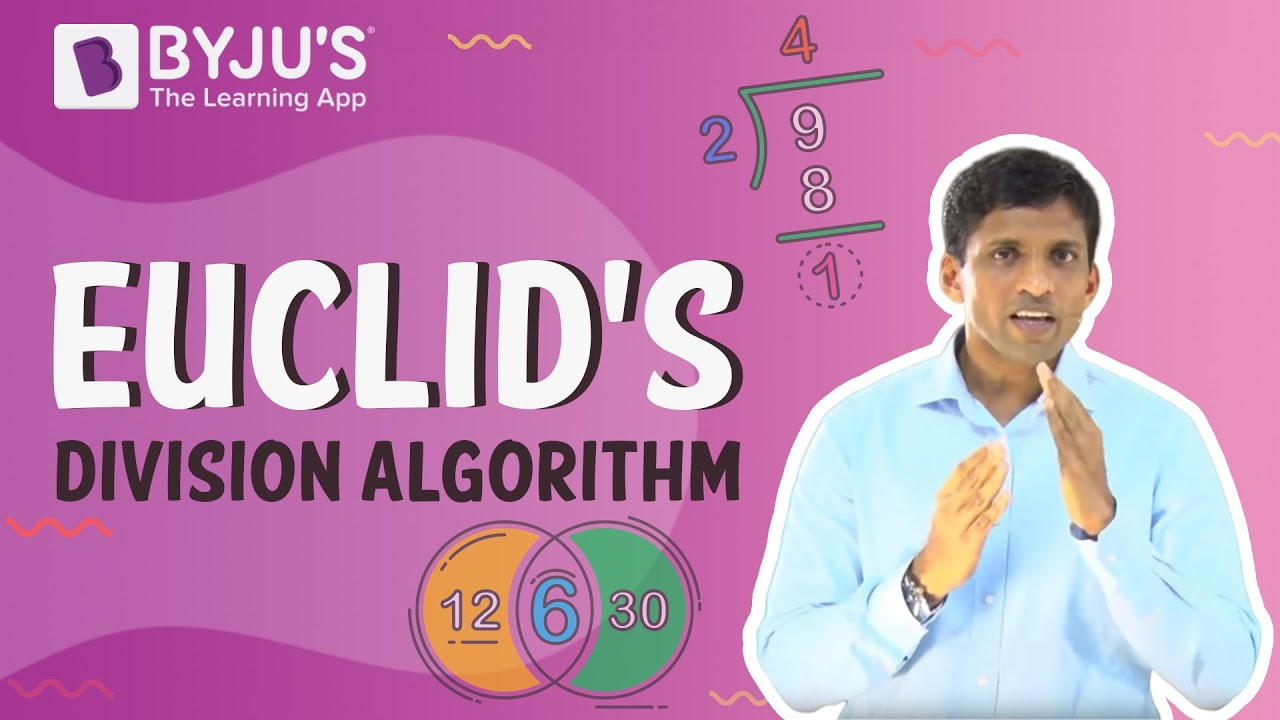# Show that every positive integer is either even or odd.

Let us assume that there exists the smallest positive integer that is neither odd nor even, say n. Since n is the least positive integer which is neither even nor odd, n – 1 must be either odd or even.

Case 1: If n – 1 is even, n – 1 = 2k for some k.

But this implies n = 2k + 1 this implies n is odd.

Case 2: If n – 1 is odd, n – 1 = 2k + 1 for some k.

But this implies n = 2k + 2 (k+1) this implies n is even.

In both ways we have a contradiction.

Thus, every positive integer is either even or odd

Check out the video below for more information on Euclid’s division algorithm### Refer more(27)(2)